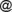# Issue:Topologies generated by intuitionistic fuzzy numbers

 shortcut http://ifigenia.org/wiki/issue:nifs/26/1/36-45
Title of paper: Topologies generated by intuitionistic fuzzy numbers
Author(s):
 R. Santhi Department of Mathematics, N.G.M., College, Pollachi, Tamilnadu, India santhifuzzyyahoo.co.in E. Kungumaraj Department of Mathematics, Sri Krishna Arts and Science College, Coimbatore, Tamilnadu, India kungum99522gmail.com
Published in: Notes on Intuitionistic Fuzzy Sets, Volume 26 (2020), Number 1, pages 36–45
DOI: https://doi.org/10.7546/nifs.2020.26.1.36-45
Abstract: In this paper we have introduced a method to generate the topologies using the intuitionistic fuzzy numbers, which is the extension work of the topologies generated by the fuzzy numbers. For this we have used (α,β)-cut of intuitionistic fuzzy number, triangular intuitionistic fuzzy number and trapezoidal intuitionistic fuzzy numbers.
Keywords: Fuzzy number, Triangular fuzzy number, Trapezoidal fuzzy number,α-cut of fuzzy numbers, Intuitionistic fuzzy number, Triangular Intuitionistic fuzzy number, Trapezoidal Intuitionistic fuzzy number, (α,β)-cut of intuitionistic fuzzy numbers.
AMS Classification: 03F55, 54A05.
References:
1. Atanassov, K. (1986). Intuitionistic fuzzy sets, Fuzzy Sets and System, 20 (1), 87–96.
2. Atanassov, K. (2012). On Intuitionistic Fuzzy Sets Theory, Springer, Berlin.
3. Burillo, P., Bustince, H. & Mohedano, V. (1994). Some definitions of intuitionistic fuzzy number, Fuzzy based expert systems, fuzzy Bulgarian enthusiasts, Sofia, Bulgaria, 1994, 28–30.
4. Klir, G. J., & Yuan, B. (1995). Fuzzy Sets and Fuzzy Logic, Theory and Applications, Prentice Hall, Inc., Upper Saddle River.
5. Padmapriya, R., & Thangavelu, P. (2014). Topologies Generated by the α cuts of a Fuzzy set, International Journal of Mathematical Archive, 2, 1–10.
6. Padmapriya, R., & Thangavelu, P. (2015). Characterization of Nearly open sets using Fuzzy sets, Global Journal of pure and applied Mathematics, 11, 18–20.
7. Padmapriya, R., & Thangavelu, P. (2015). Topologies Generated by the Fuzzy numbers, International Journal of Engineering Trends and Technology, 4, 243–246.
8. Sharma, P. K. (2011). α,β-Cut of Intuitionistic fuzzy modules, International Journal of Mathematical Sciences and Applications, 1 (3), 1489–1492.
9. Shaw, A. K., & Roy, T. K. (2012). Some Arithmetic operations on Triangular Intuitionistic Fuzzy number and its applications on reliability evaluation, International Journal of Fuzzy Mathematics and Systems, 2, 363–382.
10. Zadeh, L. (1965). Fuzzy sets, Journal of Information and Controls, 8, 338–353.
Citations:

The list of publications, citing this article may be empty or incomplete. If you can provide relevant data, please, write on the talk page.